# 抱歉，您的浏览器无法访问本站

## 物质结构

### 原子结构

#### 核素、同位素

• $\alpha$粒子：反射出$^4_2\text{He}$即两中子两质子。

• $\beta$粒子：反射出$^1_0 n$，即一中子。

#### 核外电子

##### 层数

1 2 3 4 5 6 7
$\text{K}$ $\text{L}$ $\text{M}$ $\text{N}$ $\text{O}$ $\text{P}$ $\text{Q}$

### 化学键

#### 共价键

##### 共用电子对

A：哎，那$\text{H}$，我们一起用一对电子吧。你我分别出$1\text{e}^-$。

B：好啊好啊，就让这对电子绕着我们一起转，我们假装达到相对稳定不就行了？

##### 结构式与电子式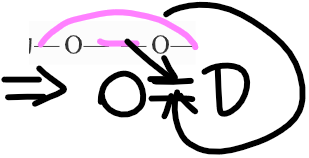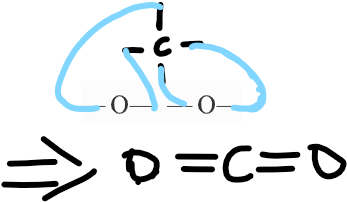## 元素周期律

### 简介

$\text{VIIA}$族为卤族，其中的元素为卤素。

### 金属活动性与非金属活动性（还原性与氧化性）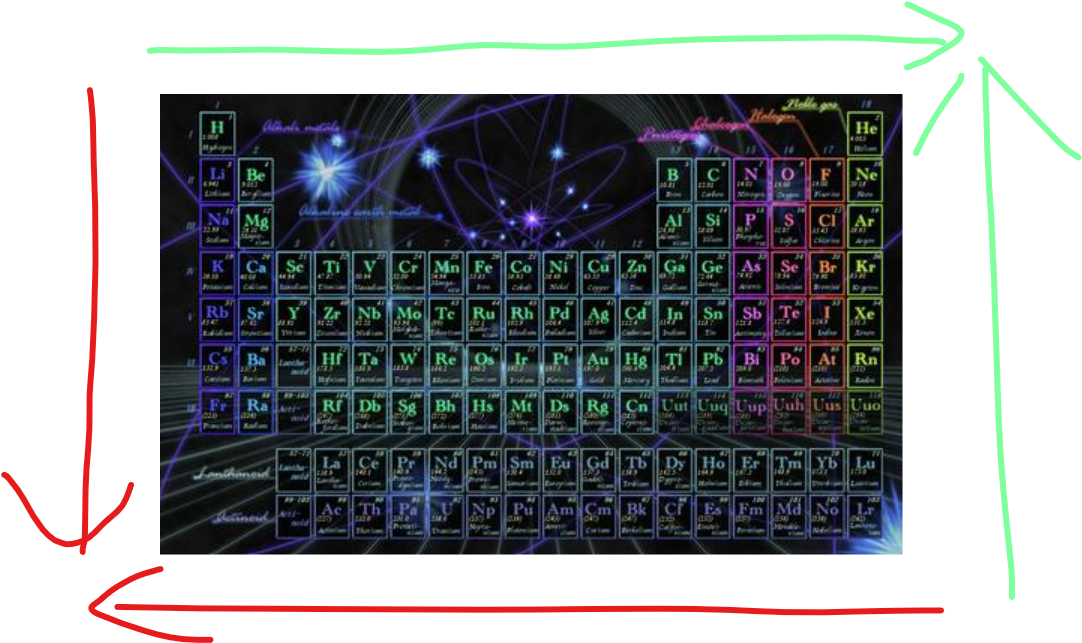## 氧化还原反应

### 双线桥和单线桥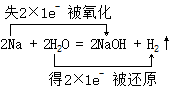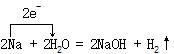### 氧化还原反应化学方程式的配平

#### 普通方程式

$\text{K}$$+1,+1$$+1$$0 \text{I}$$-1$$0$$+1$
$\text{Mn}$$+7$$+2$$-5 \text{O}$$-2,-2$$-2,-2,-2$$0$
$\text{H}$$+1$$+1$$0 \text{S}$$+6$$+6$$0$

$5\text{I}_2$有$10$碘原子，因此$\text{KI}$配$10$。

$2\text{MnSO}_4$有$2$锰原子，因此$\text{KMnO}_4$配$2$。

#### 离子方程式

$\text{Cl}$ $+5,-1$ $0$ $-5,+1$
$\text{O}$ $-2$ $-2$ $0$
$\text{H}$ $+1$ $+1$ $0$